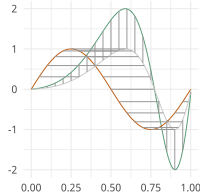# Elastic GLM Regression¶

Warping Invariant GML Regression using SRSF

moduleauthor:: Derek Tucker <jdtuck@sandia.gov>

class elastic_glm_regression.elastic_glm_regression(f, y, time)[source]

This class provides elastic glm regression for functional data using the SRVF framework accounting for warping

Usage: obj = elastic_glm_regression(f,y,time)

Parameters: f – (M,N) % matrix defining N functions of M samples y – response vector of length N time – time vector of length M alpha – intercept b – coefficient vector B – basis matrix lambda – regularization parameter SSE – sum of squared errors

Author : J. D. Tucker (JDT) <jdtuck AT sandia.gov> Date : 18-Mar-2018

calc_model(link='linear', B=None, lam=0, df=20, max_itr=20, smooth_data=False, sparam=25, parallel=False)[source]

This function identifies a regression model with phase-variability using elastic pca

Parameters: link – string of link function (‘linear’, ‘quadratic’, ‘cubic’) B – optional matrix describing Basis elements lam – regularization parameter (default 0) df – number of degrees of freedom B-spline (default 20) max_itr – maximum number of iterations (default 20) smooth_data – smooth data using box filter (default = F) sparam – number of times to apply box filter (default = 25) parallel – run in parallel (default = F)
predict(newdata=None, parallel=True)[source]

This function performs prediction on regression model on new data if available or current stored data in object Usage: obj.predict()

obj.predict(newdata)
Parameters: newdata (dict) – dict containing new data for prediction (needs the keys below, if None predicts on training data) f – (M,N) matrix of functions time – vector of time points y – truth if available smooth – smooth data if needed sparam – number of times to run filter
elastic_glm_regression.rand(d0, d1, ..., dn)

Random values in a given shape.

Note

This is a convenience function for users porting code from Matlab, and wraps random_sample. That function takes a tuple to specify the size of the output, which is consistent with other NumPy functions like numpy.zeros and numpy.ones.

Create an array of the given shape and populate it with random samples from a uniform distribution over [0, 1).

d0, d1, …, dn : int, optional
The dimensions of the returned array, must be non-negative. If no argument is given a single Python float is returned.
out : ndarray, shape (d0, d1, ..., dn)
Random values.

random

>>> np.random.rand(3,2)
array([[ 0.14022471,  0.96360618],  #random
[ 0.37601032,  0.25528411],  #random
[ 0.49313049,  0.94909878]]) #random